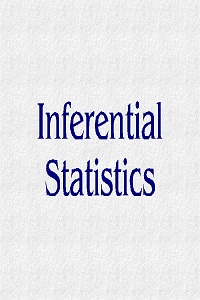advertisement

# Chapter 9.1: Inferential Statistics

### IntroductionSince the purpose of this text is to help you to perform and understand research more than it is to make you an expert statistician, the inferential statistics will be discussed in a somewhat abbreviated manner.  Inferential statistics refer to the use of current information regarding a sample of subjects in order to (1) make assumptions about the population at large and/or (2) make predictions about what might happen in the future.  The basic statistical methods explained in the previous chapter are used a great deal in inferential statistics, but the data is taken a step further in order to generalize or predict.

We can easily determine the mean of a known sample of subjects by adding up all of their scores and dividing by the number of subjects.  The mean of a sample is therefore a known variable.  To determine the mean of the population that has not been testing or to predict the mean of a test that has not yet been taken requires the researcher to make assumptions because these variables are not known to us.  The goal of inferential statistics is to do just that – to take what is known and make assumptions or inferences about what is not known.  This chapter will focus on the basic statistical procedures used for various types of data and will conclude with an explanation of how this data is used to estimate errors and make inferences.